# The Evaluation of Wind Energy Based on the Inherent Nature of Wind Speed Assessment at Fujairah (UAE)

The Evaluation of Wind Energy Based on the Inherent Nature of Wind Speed Assessment at Fujairah (UAE)
Department of Electrical Engineering, College of Engineering and Technology, University of Science and Technology of Fujairah, POB 2202, Fujairah, UAE

Corresponding Author Email:
a.abdulmajid@ustf.ac.ae
Page:
121-130
|
DOI:
https://doi.org/10.18280/i2m.200301
2 April 2021
|
Accepted:
5 June 2021
|
Published:
30 June 2021
| Citation

OPEN ACCESS

Abstract:

The aim of this paper is to determine the probability of wind speed and extracted energy at Fujairah site (UAE), based on the assessment of wind characteristics, that has been conducted with wind speed and direction measurements for over a year period (Nov. 2019- Mar. 2021), using Fast Fourier Transform, wavelet and regression analyses. Being highly Weibull, the scale and shape factors of the probability distribution function are evaluated from the mean wind speed, and at different heights, as well the probabilities at these heights. The focus of the study was on the heavily fluctuating and random nature of wind regime, during November – March, that is visible in the coefficients of the signals details since this is the period when heavier wind speeds occur, whereas a relatively calm weather is experienced during the year. The probability of wind speed, power density and extracted energy are evaluated for the mean wind speed, and for the most accessed (mode) speed and speed-rmc. The wind speed pattern is largely Weibull, with shape factor of 1.25. The scale factor is evaluated to be 2.2 m/s from the velocity occurrence probability. With mean wind speed of around 4 mph, it is suggested that the lack of wind speed can be aggregated with the penetration of other renewable energy systems such as hybrid solar, wind, PV and battery storage banks. With these hybrid systems, the diurnal and turbulence effects on wind speed harvesting, can also be compensated for to reduce wind speed variability, which depends on the daily and seasonally load demand pattern at site.

Keywords:

energy probability, Fourier decomposition, power spectrum, wavelet coefficients, Weibull distribution constants

1. Introduction

Wind speed forecasting is essential in the technical and economical assessments of harvesting wind power and installing wind turbines. Many attempts are applied to predict short and long period weather conditions using different methods such as statistical measurements, mathematical tools, and waveform analysis, whereby a statistic model  based on time series of wind power, integrated with both ANN predictions and sensitivity analysis, has been carried out. A comparison between a mathematical and physical prediction of short-period forecasting is made based on 30 years review . A waveform analysis based on evaluating the Weibull parameters of the wind signal, using several methods , such as, empirical (EM), energy pattern (EPFM), maximum likelihood (MLM), modified maximum likelihood (MMLM), as well as a graphical (GM) method.

To predict the wind speed, the Fast Fourier Transform (FFT) tool, which is based on digital Fourier transform (DFT), is used to find power spectral density of a signal. The Matlab function of FFT, is considered, to be one of the most widely implemented tool to analyze wind speed [4, 5], by extracting the frequency contents of the signal from time domain measurements. This would provide useful information about the wind speed signal, a normally random meteorological parameter which varies with time and location, that makes its prediction difficult in terms of both time and spatial domains. The inverse Fourier series is a method used to generate time histories of data, though without reflecting them to more details . Yet earlier work by Catalão et al. , studied wavelet coherence, wavelet cross correlation and the cross-correlation coefficients of spectral wavelet as functions of the equivalent Fourier period.

For better wind power utilization, there is the need to understand wind characteristics in time and spatial domains. Long and short periods of wind forecasts are important to design capacities of wind turbines and wind farms. Time series of wind speed modeling are determined, based on the assumption that influencing factors are considered concurrently at process occurrence. Such wind speed characteristics are readily available for worldwide sites, with the employments of statistical, empirical, mathematical, physical time series evaluation with Matlab platform, such as Fourier transform, wavelet transform, parameters fluctuation analysis, artificial intelligent and neural networks, autoregression and the like.

Abrupt changes in the wind speed waveform, such as transient variations, drifts, and sudden noise, are usually the most informative part of the waveform and hence, it is needed to analyze such physical superimposed effects on signal. To understand these characteristics, the wavelet analysis methods use longtime measured periods to analyze precise low-frequency information, whereas shorter periods assist to get high-frequency information.

The wavelet tool is traditionally used as a time frequency technique that decomposes signals into different frequency segments, with scale matched resolutions . It allows the use of long-time signals to focus on low frequency data information, by decomposing signals in into detailed localized information with related approximations, termed as scale and position values. The detailed data are termed as low scale data with high frequency components, whereas the approximated data parts are termed as high scale with low frequency components. The applications of wavelet analysis on wind speed signals were performed by many authors . Other applications that have been carried on, were tropical convections , monsoon phenomena , turbulence characteristics , just to name a few. In recent years, wavelet analyses have been implemented extensively to analyze the measured wind speed on structures, as a vibrating signal .

The probability density function (PDF) of the wavelet has been performed  to show that the PDF of a wavelet coefficients are deviated from the normal (Gaussian) distribution, although the power spectrum analysis agreed with what is termed Kolmogorov’s law. Chellali et al.  studied the stochastic and cyclic behavior of wind speed by fitting wind speed data with the Weibull pattern.

The extraction of wind power at different heights, based on site measurements  explored efficient selections of wind turbine tower heights. We believe that such selection of heights depends on wind distribution function too. An earlier comparison survey on energy extraction of different turbines, in low wind speed areas  is useful for this work due to the low-speed profile at Fujairah site. A similar objective to this work, is to relate extracted energy based on statistical Weibull distribution [18-20].

The main object of this work is two folded; first, is to quantify the variability nature of the wind by employing Fast Fourier Transform, power spectrum and wavelet tools. These tools are useful in quantifying highly fluctuating nature of wind speed at Fujairah area. Other periods with large fluctuations of both wind speed and direction, are focused on and analyzed for data comparison and justification. We, then correlate the Weibull constants of the wind distribution function, such as scale and shape factors, with the readily measured mean wind speed.

The second object of study is to predict possible extracted energy site, based on wind speed findings. In this context, we suggest that for such low mean speed average at Fujairah site, it is recommended to incorporate hybrid renewable energy systems with appropriately sized storage systems, such as wind-solar-battery or micro hydro storage systems, to aggregate and compensate for any power loss. With these hybrid systems, the diurnal and turbulence effects on wind speed harvesting, can also be compensated for to reduce wind speed variability, depending on the daily and seasonally load demand pattern at site.

2. Fujairah Site Description

Table 1 features Fujairah-UAE site data, which is located at located NE of the emirates, on Oman gulf coastline. The site is affected by dominantly western winds in winter and eastern winds in summer. Table 1 summarizes the main characteristics at Fujairah site.

The annual mean wind speed and direction are measured every 10 seconds with sensor located at 10 m above ground level. Data are logged and recorded together with other meteorological parameters, such as temperature, pressure, humidity, solar, etc. on SD card. Figure 1 shows the annual wind speed measured every 10 sec, but due to the calm nature of wind speed, signal data were averaged over a day. Hence, less occasionally, sporadic gusts that are blown a few times a year, each lasting for couple of hours, are eliminated and can be considered as noise, but we shall focus on assessing this confined period later in this study. Day 1 is on February 20th, 2020. It can be seen from graph that wind speed is maximum during the November - March period.

Table 1. Wind characteristics at Fujairah site

 Latitude (deg N) 25007’N Longitude 56018’E Mean wind speed at 10 m 4.072664 mph Mean wind direction 182.4651° Average temperature 28℃ Mean pressure 900-1100 m bar Relative humidity 50-100% Air density 1.188 kg/m3 Terrain flat land Obstacles Hills Surface roughness class 0.5 Sa

## 1.png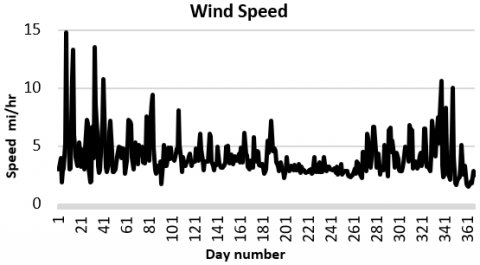Figure 1. Wind speed measurements, over the period from Nov. 2019 and Feb. 2021, yet only one year of data is displayed. The wind speed is in mph

## 2.png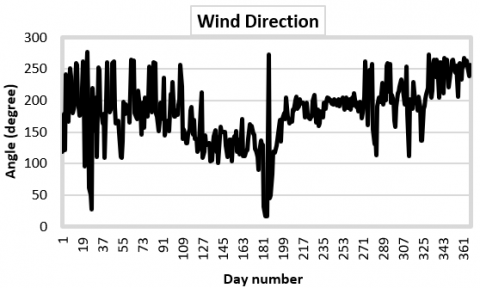Figure 2. Wind direction measurements, over the period from Nov. 2019 and Feb. 2021. The wind direction is in degrees, North referenced

The fluctuating nature of the site wind is highly random and difficult to predict in time and spatial domains, yet by focusing on windy periods, a comparable study is focused on the inherent nature of wind speed and direction. On the other hand, Figure 2 shows the annual wind direction measured over the same period along with wind speed measurements. It can be seen from figure that heavy fluctuations in wind direction occurs during February-March, in which it is mostly northern direction, whereas in summer it tends to be southern. Several parameters are affecting wind speed and direction, such as the heat convection and temperature, pressure, relative humidity, air density, as well as Fuhairah site terrain and surrounded obstacles. In this respect, the wind direction is a supplementary information of the wind nature at site, that does not constitute large information about wind speed prediction and extracted energy.

The average annual wind speed is 4.0726 mph, with maximum speed of 14.8175 mph during this confined period. The annual statistics of wind speed measurements are shown in Figure 3 with both histogram and cumulative histogram, whereas Figure 4 shows the histogram for the wind direction.

## 3.png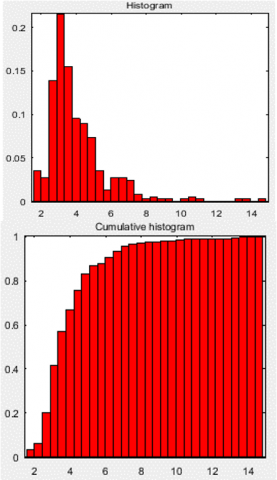Figure 3. Wind speed histogram, and cumulative histogram (extracted from Matlab). Most of variations occur in beginning of graph at low frequencies

It can be noticed that wind speed is concentrated around 2-5 mph, whereas wind direction is mostly southern to be in the range of 180°-210°, with a spike around 300°, that’s NW in direction.

## 4.pngFigure 4. Wind direction histogram (extracted from Matlab). Most of the variations occur in the end of graph at high frequenceis

3. Mathematical Background

Matlab signal analysis tool is used to determine the power spectral density by employing the Fast Fourier Transform algorithm, to determine the Discrete Fourier Transform (DFT) that transforms the time domain signals to frequency domain components, describing N-point sequence x(n) as,

$X(k)=\sum_{n=0}^{N-1} x(n) W_{N}^{n-k}$      (1)

with $\mathrm{W}_{\mathrm{N}}=\mathrm{e}^{-\mathrm{j} 2 \pi / \mathrm{N}}$, and $0 \leq \mathrm{k} \leq \mathrm{N}-1$. The wavelet analysis is used to determine the accuracy and regression of wind speeds, by decomposing the signal into detailed and approximation components. The detailed part reflects the low scale, high frequency components whereas the approximation part describes the high scale, low frequency components of the measured signal. Matlab wavelet toolbox is employed to analyze the oscillatory nature of signal with the following mathematical outlines

$\int_{-\infty}^{+\infty}|\psi(t)|^{2} d t<\infty$      (2)

$\int_{-\infty}^{+\infty} \psi(t) d t<0$      (3)

$\int_{-\infty}^{+\infty} \frac{|\psi(\xi)|^{2}}{|\xi|} d \xi<\infty$      (4)

$\psi_{a, b}(t)=a^{-0.5} \psi\left(\frac{t-b}{a}\right), a>0$      (5)

$T_{\psi} f(a, b)=a^{-0.5} \int_{-\infty}^{+\infty} f(t) \psi\left(\frac{t-b}{a}\right) d t$      (6)

where, $T_{\psi} f(a, b)$ is the wavelet transform of function f(t), termed as $<\left(f, \psi_{a, b}\right)>$. This wavelet transform decomposes a signal into several portions of coefficients, containing information about the characteristics of signal at different scales, in which information about sudden and abrupt changes, periodicity or seasonality can be extracted. The signal can be decomposed into two parts called approximation and details.  The approximation reflects the low-frequency components of the signal, whereas the details are the high frequency, lower scale signal components. We use Matlab platform to detect any discontinuities, prompt changes, long and short-term evolution of signal with meteorological parameters at the site of Fujairah.

4. Wind Characteristics Assessment

4.1 Assessment with FFT

FFT is used here being useful to extract frequencies in a stationary or transient signal over the entire time series. FFT analysis is carried out using Matlab function fft(x,n) to return the n-point DFT of the annual wind speed signal. Figure 5 is showing the FFT power spectrum density (PSD) of the measured wind speed, representing the energy with frequency, sometimes referred as periodogram, in which the horizontal ais is the normalized frequency in x π rad/sample, and the vertical axis is power/frequency in dB/(rad/sample). Figure 6 is the PSD of wind direction. The site power spectra are showing the low frequency localized around 0.05π radians per sample, with low power throughout the spectrum, which implies on the wind direction too. In general, this makes Fujairah site is not suitable for a major wind harvesting, although measurements were logged on 10 m. It is considered to classified as wind power class 2, with wind power density of 100 W/m2 .

## 5.png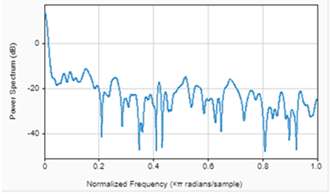Figure 5. Wind speed power spectrum with the normalized frequency (extracted from Matlab). Power spectrum in dB, whereas frequency is in radians per sample

The wind direction, on the other hand, is projected to be either north-western or south-eastern, with frequent swinging within 90°, almost daily. This indicates the turbinal nature of the wind characteristics due to the site outskirt on the seacoast, surrounded by hills. Due to these circumstances, it is worth mentioning, that the location of data logging is critical, and hence, several logging sites are needed to consolidate results.

As depicted from Figure 5, the Fourier coefficients during winter (Nov.-Mar.) are large, confined, and non-scattered, whereas during other times in summer, the Fourier coefficients are small and more scattered. This is due to the rapid heat convection process. To evaluate the periodicity of wind speed data, several Periodogram plots are carried out, such as the single-sided amplitude spectrum of Fourier power, which is the sum of square of absolute values of Fourier coefficients, as shown in Figure 7, in which the auto correlation function of wind time series against number of lags. It is clear, that this curve is not symmetrical, so wind series data is not directly predictable.

## 6.pngFigure 6. Wind direction spectrum, in dB with normalized frequency in radians per sample (extracted from Matlab). Several spikes in power at different frequencies

## 7.png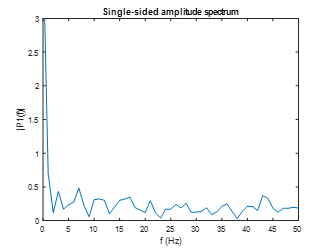Figure 7. Single-sided spectrum (extracted from Matlab) showing wind speed amplitude spectrum with frequency in Hertz. It indicates relatively calm speed

To have a close look at the periodicity peaks, wavelet transform is introduced in which the Fourier power peaks are observed with their corresponding frequencies. By projecting the frequency ranges corresponding to maximum power, the periodicity can be determined, to be different at different seasons. With the scaling function, it is further possible to zoom into signal to extract some valuable and distinct changes over the summer and winter periods.

4.2 Wind speed assessment with wavelet decomposition

Wavelet decomposition of the wind speed signal into 4 levels, together with threshold values, is depicted in Figure 8. Due to low wind regime at site, there is no need for more decomposition levels due to low signal amplitudes at higher frequencies. To refine the pattern of these levels, values of noise thresholds generated by decomposition, are displayed by Matlab wavemenue function. While FFT produces the average of spectral coefficients, independent of time, in order to identify the dominant frequencies in a signal, the wavelet transform provides information on time frequency localization of signal duration. This is depicted in Figure 8.

## 8.png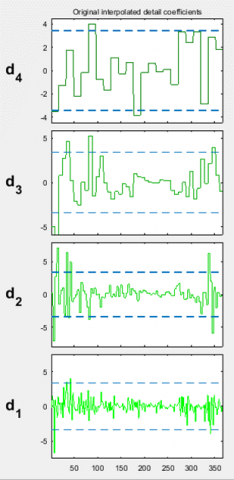Figure 8. Wind speed wavelet coefficients, with four threshold details for a period of a year (extracted from Matlab). Threshold values of ± 3 are displayed in all levels

For a long-term wind speed signal, the decomposition of signal at several frequencies, is useful to understand the inherent characteristics of wind. Matlab wavelet toolbox provides essential information of signal time-frequency decompositions at different levels, that is posing needed knowledge about the inherent characteristics of the wind signal.

In this study, we assumed a simple Haar wavelet to analyze and differentiate the summer-winter periods of signal wind speed. Both data are decomposed by Haar wavelet up to the 4th level using menu wavemenu in Matlab command prompt and plotting them.

The four levels depicted in Figure 8 are the decomposition steps from d4 to d1, showing the details of the decomposed signals of the raw signal in the entire period of one year. In each level, the low frequency of the previous level is decomposed into low and high adjacent bands of frequencies, as low pass (LP) and high pass (HP) filter pairs, each with half of the bandwidth of the previous frequency scan range. Each filter band is decimated by a factor of 2 to preserve the same bit rate of the original signal. Figure 8 shows the wavelet decomposition analysis, with four levels, using simple Haar wavelet.

The approximation signal, representing the low frequency of the amplitude resembled raw signal, is shown in Figure 9. It shows the temporal wind turbulences, typically occurring in the February-March period, when atmospheric temperature is changed quickly.

## 9.pngFigure 9. Approximate coefficient of the wind speed waveform, over the period of a year. It summarizes wind speed characteristics over the entire period of over a year

The coefficients of low-high filter effects are readily computed by Matlab wavelet toolbox. It is evident from Figure 9 that the ‘a4’ signal takes the shape of the raw signal with similar peaks at different periods of the year. Similarly, the threshold level of each decomposed level of the wind is shown.

Figure 10 shows the histogram of the coefficients of the approximation waveform, for the focused period between November-April, as most of the wind activity occurs in this period, whereas Figure 11 shows the histogram of the detail level 1, during the same period. It shows larger coefficient values, with smaller standard deviation.

## 10.png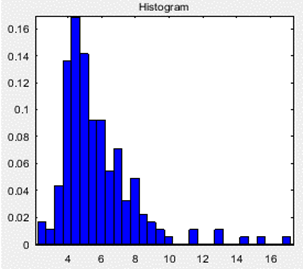Figure 10. Histogram of the wind speed coefficients of the approximation level (extracted from Matlab)

## 11.pngFigure 11. Histogram of the wind speed coefficients of the detail at level 1

## 12.pngFigure 12. The compressed signal, original coefficients, and coefficients thresholds (extracted from Matlab). The coefficients levels are drawn against wind speed waveform samples, over a period of a year

Both figures show temporal turbulence effects that lasts only for a month, due to the seasonal temperature change from winter to summer, and vice verse.

The mean values of the synthesized and original signals are found to be equal with normalized error between them at level 1, found to be 4.9175e-14. The synthesized signal is reconstructed from the decompositions of the original signal, according to wavelet type name and levels.

Table 2 summarizes assessment of the wind speed residual coefficients, depicted from the data of wavelet coefficients of Figure 8. Figure 12 depicts the compressed signal of the original raw signal over the entire period of wind measurements, together with the 4 stacked coefficient levels shown earlier in Figure 8.

Table 2. Wind speed residual assessment

 Mean 0.531 e-05 Medan -0.1781 Mode -0.2372 Maximum 5.77 Minimum -3.190 Range 8.966 Standard Dev. 1.287 Medan Abs. Dev. 0.6037 Mean Abs. Dev. 0.912 L1 norm 334.7 L2 norm 24.63 Max norm 5.77

The upper part depicts noise effect with both, the raw signal and the compressed denoised signal. The middle part shows wavelet decomposition coefficients through 4 levels, whereas the lower part of the figure shows the same threshold coefficients after de-noising the signal, which helps in identifying samples of high wind speeds. Table 2 summarizes the results of residual assessment.

4.3 De-noised wind signal assessment

Figure 13 shows both the compressed signal and the de-noised signal. It can be seen, that comparing the two signals, does not show more discrepancies, as less than 10% of peak amplitudes are affected by noise.

## 13.png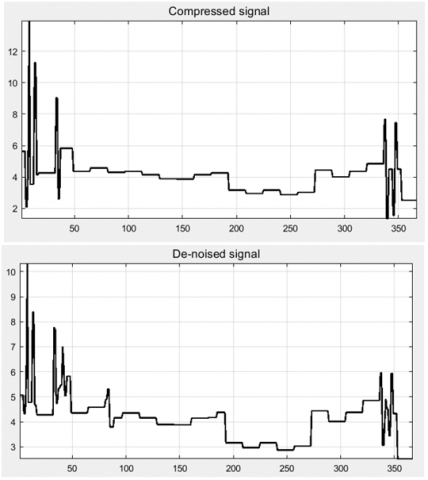Figure 13. Compressed and de-noised wind speed signal, which indicates around 10% noise

It is apparent that starting from February (February 20th =1st day), there is a peak in wind speed throughout till end of March, and again during November-December period. This phenomenon is due to the temporal thermal change in the Fujairah site airs.

4.4 Signal residual assessment

## 14.pngFigure 14. Wind speed residual statistics, showing histogram, cumulative histogram, autocorrelation as well as FFT spectrum (extracted from Matlab)

## 15.png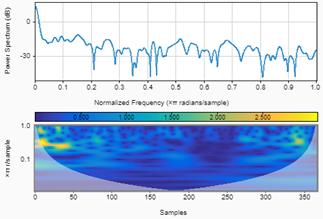Figure 15. Wind speed scalogram of the wind signal over a year period, showing power spectrum and scalogram (extracted from Matlab)

## 16.png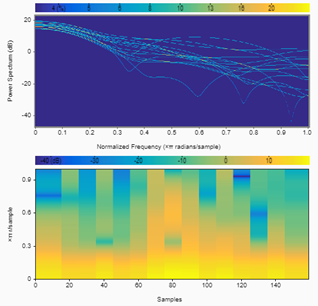Figure 16. Wind speed spectrogram for the confined period of 4 months when speed activity and variation is maximum (extracted from Matlab)

## 17.png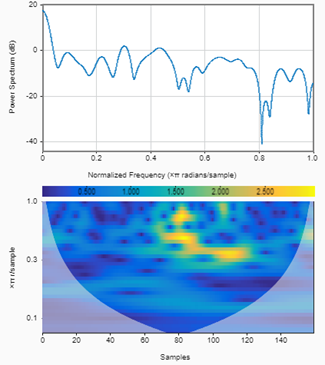Figure 17. Wind speed confined scalogram together with power spectrum over 4 a period of 4 months, with maximum variations (extracted from Matlab)

The residuals of signal, which is the difference between the estimated and observed values, are used in the regression analysis. Figure 14 shows the assessed residuals of the measured signal, in which there is a rise at the wind speed data during the 10-100 days, and again during the 280-360 days, with sharp variations at different days. The histogram and cumulative histograms are also shown in Figure 14, together with the autocorrelation of the wind speed and FFT energy spectrum at the initial rise of energy spectrum data.

These results can also be seen in the scalogram of the wind speed, with the light, yellow regions in the beginning and end of the entire annual spectrum. This is depicted in Figure 15.

Zooming into the confined 150 days region, where wind speed activity is highest, we can recognize the power spectrum with the normalized frequency, as shown in Figure 16. It can be seen, that during temperature prompt changes from autumn to winter, and winter to spring, clear changes occur in both wind speed and direction, as depicted in both power spectrum with normalized frequencies, as well xtti per sample plots.

Figure 17 shows the scalogram of the wind speed at the confined 150 days region (Nov-April). Yellow colored regions reflect the sharp speed increase during February-March.

Prediction of wind speed at Fujairah site is not simple due to the random turbulence effects due to the prompt temperature change in late autumn and early spring of the year. The wind direction, on the other hand, is predictable due to the site outskirt, being on the sea gulf coast and located among hilly regions.

5. Evaluation of Wind Energy

The second major part of this work is to relate and estimate the extracted energy from available wind speed. Based on the earlier assessment of wind characteristic, we shall estimate the energy that can be extracted, and the possible arrangements and solutions to enhance the output power and energy from the relatively low and highly variable wind speed at site.

The probability of wind speed occurrence P, (% of hours/year per mph), is normally expressed by the Weibull distribution function , as

$P(v, k, c)=\frac{k}{c}\left(\frac{v}{c}\right)^{k-1} \exp \left[-\left(\frac{v}{c}\right)^{k}\right]$     (7)

where, v is wind speed, c is a scale parameter that controls the mode of most probable speed. k is a shape parameter that controls the shape of the distribution, such as Exponential, Gaussian or Weibull distributions. As the logged wind speed resembles a sharp Weibull pattern, a value of k =1.25 is chosen. It can also be seen that the distribution is from Figure 3, that the scale parameter c is ranging between 2 and 2.5, and we can estimate it by integrating (7) over the whole range of wind speeds, as

$V_{\text {mean }}=\int_{0}^{\infty} v \frac{k}{c}\left(\frac{v}{c}\right)^{k-1} \exp \left[-\left(\frac{v}{c}\right)^{k}\right] \mathrm{d} \mathrm{v}$     (8)

By substituting Vmean = 1.8 m/s (4.072 mph as the measured speed), a value of c = 2.2m/s is calculated. Also, it can be seen from Figure 3 that the mean wind speed, mostly harvested, normally termed as Vmode, is 1.33 m/s (3 mph as logged).

From Table 1, the air density at the site location = 1.188 kg/m2 and by substituting these values of c, h and ρ, in (7) we get a probability of occurrence of a given wind speed as expressed by the Weibull distribution, which is largely characterized by Fujairah site data.

Hence, the power density $0.5 \rho v^{3}$ can be evaluated for Vmode, Vmean and root mean cubic velocity, Vrmc., as shown in Table 3, where Vrmc is determined as,

$V_{r m c}=\sqrt{\int_{0}^{\infty} v^{3} \frac{k}{c}\left(\frac{v}{c}\right)^{k-1} \exp \left[-\left(\frac{v}{c}\right)^{k}\right] \mathrm{d} v}$      (9)

The total energy that can be extracted is calculated as the integral of the power density for all available wind speeds, over a year as,

Energy $=0.5 \rho \mathrm{v}^{3}(8760)$      (10)

This energy can be further adjusted by multiplying it with a coefficient that depends on the type of wind turbine used. Table 3 summarizes the power density and energy for each velocity.

Table 3. Power and energy against velocity

 Velocity (m/s) Probability Power density (Watt/m2) Energy (kWh/m2 /yr.) Vmean = 1.800 0.2481 3.464 30.344 Vmode = 1.333 0.2936 1.397 12.237 Vrmc = 3.157 0.1293 18.690 163.724

At lower wind speeds with v < 5 mph, the probability is not only small but highly unpredictable. The wind speed measurements were logged at 10 m height above ground level. At higher height Z2, the new wind speed v2 is related with speed v1 at Z1, as

$\mathrm{v}_{2}=\mathrm{v}_{1}\left(\frac{\mathrm{Z}_{2}}{\mathrm{Z}_{1}}\right)^{\alpha}$     (11)

where, α is wind shear exponent, that depends on Fujairah site landscape type, which is a small city with land of rough and uneven terrain, with hedgerows and hills. Hence, with a value of α=0.4 assigned for this site, would provide a mean wind speed of 5 mph at 30 m above ground level. Table 4 depicts the Weibull probability factors, k and c for different heights of wind speed. We assume k constant since wind speed pattern is highly Weibull distribution.

Table 4. Weibull probability factors

 Height (m) Velocity (m/s) k c Probability 10 2.35 1.25 2.8 0.1912 20 2.79 1.25 3.3 0.1614 30 3.14 1.25 3.7 0.1438

With the above values of v, k and c, at Fujairah site, it would be not recommended that power can be utilized with wind turbines alone but may be aggregated with other renewable systems as hybrid systems, in order to remove short term variability of power penetration. Figure 18 depicts a typical power harvesting system with hybrid solar-wind-storage power supplies. This is with reference to the work carried out , which reflects the characteristics of the solar and wind patterns at Fujairah site. It can be seen, that with such assessed wind speed curve, a wind turbine rating can be selected to accommodate all surplus energy needed by an assumed load demand, using battery or similar storages, incorporated with solar PV modules.

## 18.pngFigure 18. Proposed hybrid solar-wind-storage system for Fujairah site

It can be shown that diurnal and turbulence effects on wind speed harvesting, can be compensated with the penetration of other renewable energy systems such as solar and battery storage, since solar PV modules can supply load power LX during sun path duration GPV, while wind energy is stored with excess solar energy BX and BY, in batteries during other times. That’s , $G_{P V}=B_{X}+B_{Y}$. The rating of battery banks is related with their depth of discharge, while the PV rating is set to be the peak of load distribution waveform that accounts for the difference between day load and night load, since it is assumed that for domestic and residential applications, day load is normally higher than night load, that’s , $L_{X}=L_{D}-L_{N}$. where LD and LN are the day and night load demands, respectively. Likewise, seasonally the wind speed at Fujairah is higher than summer speed, a situation that compensates for extra needed heating energy, whereas during summer, the heavy air-conditioning load demand should be relied dominantly on the solar energy.

6. Conclusions

The first part of this work is to estimate the wind characteristics at Fujairah site. The mean wind speed at site is found to be 4.072 mph at 10 m above ground level, reaching maximum of 14.817 mph during February month, with mean absolute deviation of 9.531 e-05, whereas the maximum and minimum values are 5.77 and -3.190 respectively, and standard deviation of 1.287. The mean long-term fluctuation of wind speed is found to be within ± 10% of wind speed, whereas short term fluctuation over the confined 4 months period (Nov.-Mar.) is more than ± 20%. The spectrogram and scalogram of the wind fluctuating nature of both speed and direction are applied, to determine the time and spatial domain for the year period. The wind speed pattern is largely Weibull, with shape factor of 1.25. The scale factor is evaluated to be 2.2 m/s from the velocity occurrence probability.

The second objective of this study is to predict extracted energy, based on the inherent wind characteristics. The annual wind speed data at site, are used to evaluate the scale and shape factors of the Weibull distribution function. We assumed that Weibull factor k is unchanged with height, yet further work may consider it to be variable. The probability of wind speed, power density and extracted energy are evaluated for the mean wind speed, the mode speed, and speed root-mean-cube, as well as for different heights. Based on these data, the power density and energy are evaluated. A case example is presented to overcome outcome deficiencies, in which a hybrid system of solar, wind and an appropriately sized storage system is proposed. It shows that with proper sizing arrangement of hybrid systems, it is possible to manage energy transfer to load demand under these conditions of low wind speed regimes.

It is suggested that for future extended work, the probability distribution functions of the Weibull factors can be determined, as well as their joined probability to determine this effect on the wind speed prediction.

Acknowledgment

Amir J. Majid would like to thank Eng. Momen Odeh, m.odeh@ustf.ac.ae for prototyping the wind measuring setup, and Harris Majid, pk2_pk@yahoo.co.uk for data logging with Python.

Nomenclature
 X signal in frequency domain x signal in time domain Ta wavelet transform operator P probability k shape factor c scale factor (m/s) v velocity (m/s) V velocity root mean cube (m/s) z height (m) t time (s) a wavelet scale operator b wavelet time shift Greek symbols $\psi$ wavelet operator ζ Integration domain ρ Air density (kg/m2) α wind shear exponent Subscripts N,n,k indices
References

 Giogi, M.D., Ficarella, A., Tarantino, M. (2011). Assessment of the benefits of numerical weather predictions in wind power forecasting based on statistical methods. Energy, 36(7): 3968-3978. https://doi.org/10.1016/j.energy.2011.05.006

 Costa, A., Crespo, A., Navarro, J., Lizcano, G., Madsen, H., Feitosa, E. (2008). A review on the young history of the wind power short-term prediction. Renewable and Sustainable Energy Reviews, 12(6): 1725-1744. http://dx.doi.org/10.1016/j.rser.2007.01.015

 Hulio, Z.H., Jiang, W. (2018). Site-specific technical and economic analysis of wind power potential and energy generation using Weibull parameters. World Journal of Science, Technology and Sustainable Development, 15(1): 35-53. http://dx.doi.org/10.1108/WJSTSD-10-2016-0058

 Wirsing, K. (2020). Time Frequency Analysis of Waeley and Fourier Transform. http://doi.org/10.5772/intechopen.94521

 Alam, M.M., Rehman, S., Al-Hadhrami, L.M. (2014). Extraction of the inherent nature of wind speed using wavelets and FFT. Energy for Sustainable Development, 22: 34-47. http://dx.doi.org/10.1016/j.esd.2014.02.004

 Kitagawa, T., Nomura, T. (2003). A wavelet-based method to generate artificial wind fluctuation data. Journal of Wind Engineering and Industrial Aerodynamics, 91(7): 943-964. http://dx.doi.org/10.1016/S0167-6105(03)00037-0

 Catalão, J.P.S., Pousinho, H.M.I., Mendes, V.M.F. (2011). Short-term wind power forecasting in Portugal by neural networks and wavelet. Transform, Renewable Energy, 36(4): 1245-1251. http://dx.doi.org/10.1016/j.renene.2010.09.016

 Shinozuka, M., Deodatis, G. (1991). Simulation of stochastic processes by spectral representation. Applied Mechanics Reviews, 44: 191-204. http://dx.doi.org/10.1115/1.3119501

 Turbelin, G., Ngae, P., Grignon, M. (2009). Wavelet cross-correlation analysis of wind speed series generated by ANN based models. Renewable Energy, 34: 1024-1032. http://dx.doi.org/10.1016/j.renene.2008.08.016

 Yamada, M., Ohkitani, K. (1991). Orthonormal wavelet analysis of turbulence. Fluid Dynamics Research, 8(1-4): 101. http://dx.doi.org/10.1016/0169-5983(91)90034-G.

 Kitagawa, T., Nomura, T. (2003). A wavelet-based method to generate artificial wind fluctuation data. Journal of Wind Engineering and Industrial Aerodynamics, 91(7): 943-964. http://dx.doi.org/10.1016/S0167-6105(03)00037-0

 Weng, H., Lau, K. (1994). Wavelets, period doubling, and time–frequency localization with application to organization of convection over the tropical western Pacific. Journal of the Atmospheric Sciences, 51(17): 2523-2541. http://dx.doi.org/10.1175/1520-0469(1994)051%3C2523:WPDATL%3E2.0.CO;2

 Torrence, C., Compo, G.P. (1998). A practical guide to wavelet analysis. Bulletin of the American Meteorological Society, 79: 61-78.

 Farge, M. (1992). Wavelet transforms and their applications to turbulence. Annual Review of Fluid Mechanics, 24: 395-458. http://dx.doi.org/10.1146/annurev.fl.24.010192.002143

 Rossi, R., Lazzari, M., Vitaliani, R. (2004). Wind field simulation for structural engineering purposes, International Journal for Numerical Methods in Engineering, 61(5): 738-763. http://dx.doi.org/10.1002/nme.1083

 Tizgui, I., Bouzahir, H., El Guezar, F., Benaid, B. (2017). Wind speed extrapolation and wind power assessment at different heights. International Conference on Electrical and Information Technology, Rabat, Morocco, pp. 15-18. http://dx.doi.org/10.1109/EITech.2017.8255215

 Ani, S.O., Polinder, H., Ferreira, J.A. (2011). Energy yield of small turbines in low wind speed areas. 3rd IEEE International Conference on Adaptive Science and Technology (ICAST 2011), Abuja, Nigeria, pp. 24-26. http://dx.doi.org/10.1109/ICASTech.2011.6145160

 Aldaoudeyeh, A.I., Alzaareer, K. (2020). Statistical analysis of wind power using Weibull distribution to maximize energy yield. 2020 IEEE PES/IAS Power Africa, Nairobi, Kenya. http://dx.doi.org/10.1109/PowerAfrica49420.2020.9219829

 Mohsin, M., Rao, K.V.S. (2018). Estimation of Weibull distribution parameters and wind power density for wind farm site at Akal at Jaisalmer in Rajasthan. 3rd International Innovative Applications of Computational Intelligence on Power, Energy and Controls with Their Impact on Humanity (CIPECH), Ghaziabad, India. http://dx.doi.org/10.1109/CIPECH.2018.8724170

 Mahmood, F., Resen, A.K., Khamees, A.B. (2020). Wind characteristic analysis based on Weibull distribution of Al-Salman site, Iraq. Elsevier ScienceDirect, Energy Reports, 6(Supplement 3): 79-87. http://dx.doi.org/10.1016/j.egyr.2019.10.021

 Hodge, B.K. (2017). Wind Energy Resources, in Alternative Energy Systems and Applications. John Wiley & Sons.

 Majid, A. (2021). Economic planning of the operation of PV-connected distribution network using a probabilistic model for extending the network lifetime, International Journal of Sustainable Development and Planning, 16(1): 97-103. http://dx.doi.org/10.18280/ijsdp.160110.

 Patel, M.R. (2005). Wind and Solar Power Systems. 2nd ed., New York, Taylor & Francis.